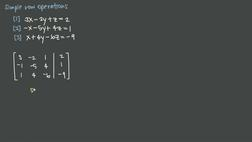PLAY PREVIEW

### Segments in this Video

#### Matrixes in Systems of Linear Equations(01:01)

FREE PREVIEW

There are three rows operations that can be used to solve a linear system: row addition, row multiplication, and row substitution.

#### Row Operations(01:48)

There are three row operations to focus on which involve switching rows. Once you have switched the first and second row, you can make any row switches needed. The matrix still represents the linear system.

#### Row Switches and Scaling(00:57)

A matrix is just a way of organizing a system of linear equations. The rows and columns of the matrix correspond to the equations, and the values in the matrix represent the coefficients of the variables in the equations. We can multiply rows by constants to scale them and we can switch the rows around to change the order of the equations, but this does not change the solution to the system.

#### Constructing a Matrix(01:26)

To multiply a matrix by a scalar, multiply each value in the matrix by the scalar.

#### Row Operations in the Matrix(01:35)

A scaling row operation is when you multiply a row by a scalar. You can do this to any row. The last row operation we want to look at is adding rows. We can add rows because when we were doing elimination to solve the system we could add equations together.

#### Constructing a Matrix(00:40)

Adding two rows of a matrix together result in a new matrix where the row operation has been performed. The original matrix is unchanged.

#### Changing Rows(02:46)

When revealing rows 2 and 3, you replace row 2 with the sum of row 2 and 3. Adding and subtracting rows are the same as scaling and adding. Multiplying a row by a negative number is the same as subtracting that row.

#### Credits(00:00)

Credits

For additional digital leasing and purchase options contact a media consultant at 800-257-5126
(press option 3) or sales@films.com.

# Simple row operations

Part of the Series : Integral Calc: Linear Algebra
 3-Year Streaming Price: \$49.95

Share

### Description

This video tutorial works through math problems/equations that address topics in Linear Algebra. This specific tutorial addresses Simple row operations.

Length: 11 minutes

Item#: BVL275886Closed Captioned